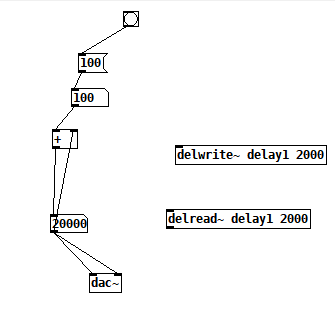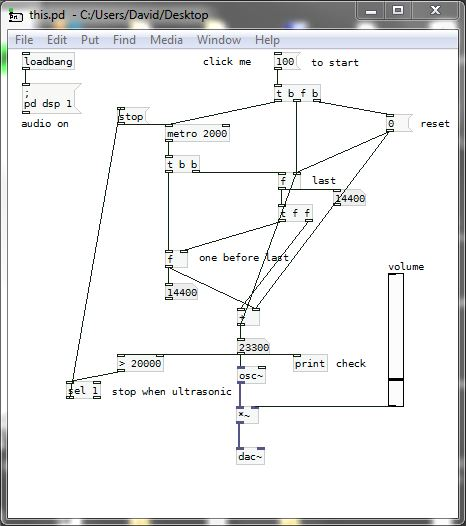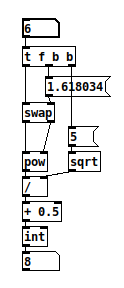• ### How to make sound with gradually increasing frequency

Hi, I want to make the sound with gradually increasing frequency.

For example

after 0s 100 Hz
after 2s 100Hz + 100Hz = 200Hz
after 4s 100Hz + 200Hz = 300Hz
after 6s 200Hz + 300Hz = 500Hz
after 8s 300Hz + 500Hz = 800Hz
....
and so on
...I tried to write code but simply didn't work
I would be very grateful if you could help me with writing code

• Posts 4 | Views 195
• @yaonway Try......... this.pdIt is using [f] ..... to store the previous values. Have a look at this to understand how.......
https://forum.pdpatchrepo.info/topic/13320/welcome-to-the-forum

Your series seems to be "frequency = sum of previous two frequencies".
It will rapidly become ultrasonic so I have put a stop once it has done so.
You can replace [osc~]...... a sine wave..... with a sound generator of your choosing.

If you actually want a smooth change arriving at the desired frequency at the end of each 2 seconds then use [line] like this........ this2.pd
David.

• @whale-av said:

If you actually want a smooth change arriving at the desired frequency at the end of each 2 seconds then use [line]

or [line~] for even more smoothness~

• @whale-av said:

Your series seems to be "frequency = sum of previous two frequencies".

So a fibonacci sequence. You can cut out the extra float, do a simple +1 count, run it through a fibo algorithm and multiply by starting frequency. fibonacci.pdTo be fair you could just do `[expr int(0.5 + pow(1.618034, \$f1)/sqrt(5))]` but I am the grumpy old anti expr fundamentalistPosts 4 | Views 195
Internal error.

Oops! Looks like something went wrong!